# Interpolation Basics

This article covers interpolation basics, and provides a numerical example of interpolation of a time signal.  Figure 1 illustrates what we mean by interpolation.  The top plot shows a continuous time signal, and the middle plot shows a sampled version with sample time Ts.  The goal of interpolation is to increase the sample rate such that the new (interpolated) sample values are close to the values of the continuous signal at the sample times .  For example, if we increase the sample rate by the integer factor of four, the interpolated signal is as shown in the bottom plot.  The time between samples has been decreased from Ts to Ts/4.

The simplest technique for interpolation is linear interpolation, in which you draw a straight line between sample points, and compute the new samples that fall on the line.  However, in many cases, linear interpolation is not accurate enough.  Other techniques involve fitting polynomials to the time function.  Here, we’ll show the power of approaching the problem in the frequency domain.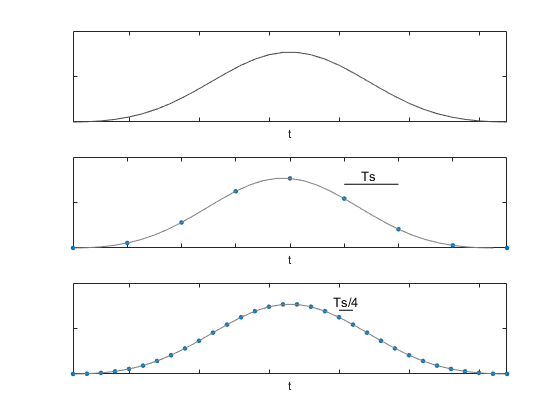Figure 1.  Top:  Continuous signal

Middle:  Signal with sample time Ts

Bottom:  Interpolated signal with sample time Ts/4

Before we describe how interpolation works, let’s look at two sampled signals and their spectra.  In the top row of Figure 2, the time signal u is sampled at 400 Hz, and its magnitude spectrum is shown on the right.  In the bottom row, the time signal x consists of every fourth sample of u, and thus has sample rate of 100 Hz.  The spectrum is again shown on the right, with the image spectrum above 100 Hz shown by a dashed line.  Note the amplitude of the spectrum is ¼ that that of u.

Now let’s look at an example of interpolation by an integer factor of four.  For the signal x just described, interpolation by four should result in the signal u.  Matlab code for the example interpolator is provided at the end of the article.

If we simply increase the sample rate of x from 100 Hz to 400 Hz, we get the signal shown in Figure 3.  Here we’ve just inserted three zeros between each of the original samples of x, a process called upsampling.  Upsampling includes scaling the amplitude of x by the upsampling ratio of 4, which gives a maximum spectrum magnitude of 1, instead of 1/4.  The spectrum of the upsampled signal xup is shown on the right.  Given that xup sample values have the same time spacing as x, the spectrum is the same as that as x, except its amplitude is a factor of 4 larger.

Now, if we compare the spectrum of xup to that of u, they match from 0 to 50 Hz, but xup has additional image spectra centered at 100 and 200 Hz.  So, it seems that we should be able to approximate u by low-pass filtering xup to attenuate the images.  If this works, we will have interpolated x by four.  A block diagram of the interpolator is shown in Figure 4.  In our case L = 4.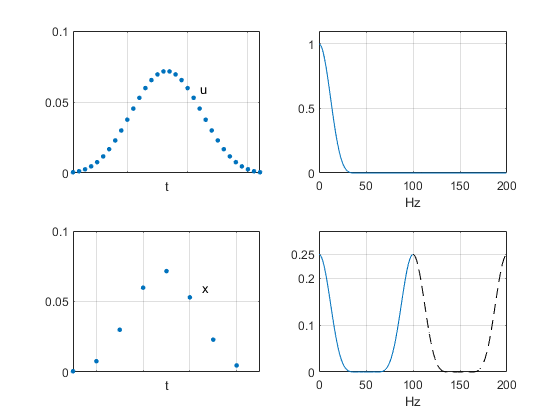Figure 2.  Top:  sampled signal u with fs = 400 Hz and its spectrum (linear amplitude scale)

Bottom:   sampled signal x with fs = 100 Hz and its spectrum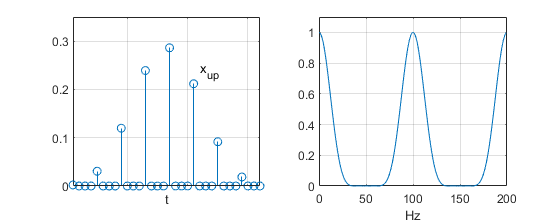Figure 3.  Upsampled signal xup with fs = 400 Hz and its spectrum (linear amplitude scale)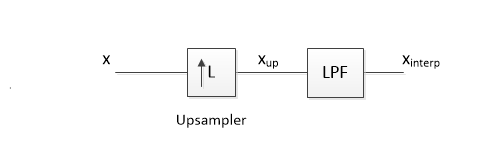Figure 4.  Interpolator block diagram (for our example, L = 4)

To design the low-pass filter, we’ll look at the dB spectrum of xup, as shown in the top of Figure 5.  We need a filter that passes the desired spectrum and attenuates the undesired images.  Thus, we have the following passband and stopband:

Passband:  0 to 36 Hz

Stopband:  66 to 135 Hz and 166 to 200 Hz

We leave the ranges from 50 to 66 Hz and 135 to 166 Hz unspecified, since the amplitude of xup’s spectrum is low in these ranges.  The Matlab code at the end of this article includes synthesis of a 41-tap FIR interpolation filter.  The filter response is shown in the middle of Figure 5.  The spectrum of xinterp is shown in the bottom plot.

The time signal xinterp is shown on the left of Figure 6, with the linear-amplitude spectrum on the right.  As hoped, xinterp resembles the plot of u in Figure 2.   We can compute the error between xinterp and u as:

% error = 100*(xinterp – u)/max(u)

The error is plotted in Figure 7.   Reflecting on how we achieved this result, it is worth noting that we performed interpolation in time by applying knowledge of the input signal’s frequency spectrum.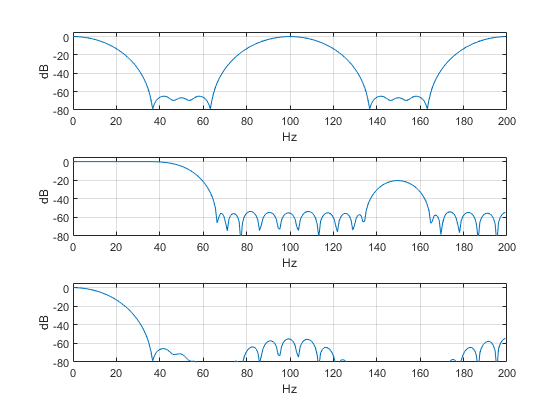Figure 5.  Top:  Spectrum of xup

Middle:  Interpolation low-pass filter response

Bottom:  Spectrum of xinterp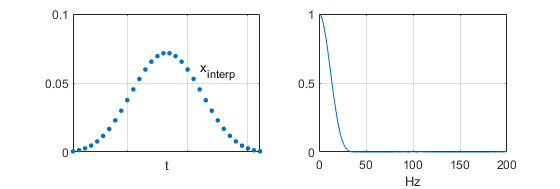Figure 6.  Left:  Interpolator output xinterp (compare to u in Figure 2)

Right:  spectrum of xinterp (linear amplitude scale)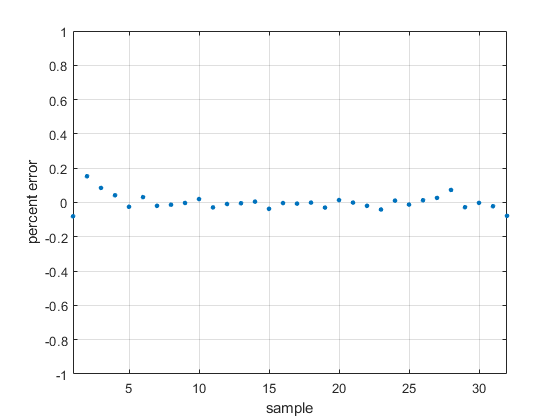Figure 7.  Interpolation percent error

## Matlab code demonstrating interpolation by four

The following code uses the same signal names as the text:

u                        signal sampled at 400 Hz

x                        interpolator input signal (formed from every 4th sample of u)

x_up                  upsampled version of x, fs = 400 Hz

x_interp             interpolation filter output, fs = 400 Hz

The signal u is a Chebyshev window function of length 32 with -70 dB sidelobes [2,3].  Other functions could be used, for example a Blackman window.  As previously discussed, the calculation of xup includes scaling by 4.  The interpolator output x_interp has length 72.  Samples 21:52 are used as an approximation of the signal u.

The interpolation filter has fs = 400 Hz and is synthesized using the Parks-McClellan algorithm (Matlab function firpm).  The coefficients are plotted in Figure 8, and the filter’s frequency response is shown in the center plot of Figure 5.  Note that while this design approach is straightforward, it does not result in the most efficient interpolator.  One alternative approach would cascade two interpolate-by-two sections, as shown in Figure 9.  This allows the use of halfband interpolation filters .  For a discussion of efficient approaches to interpolator design, see .

```%interp_demo.m  8/17/2019 Neil Robertson
% Demonstrate interpolation by 4
fs= 400;                % Hz sample rate
p= chebwin(32,70)';     % signal = Chebyshev window
u= p/sum(p);            % normalize for sum = 1
x= [u(1:4:end)];        % interpolator input signal, fs= 100
% upsampling
x_up= zeros(1,32);
x_up(1:4:32)= 4*x(1:8); % upsampled signal
%
% interpolation filter using Parks-McClellan algorithm
fn= fs/2;
f= [0 36 66 135 166 199]/fn;    % frequency vector
a= [1 1 0 0 0 0];               % amplitude goal vector
Ntaps= 41;
b= firpm(Ntaps-1,f,a);          % synthesize filter coeffs
b= round(b*2^13)/2^13;          % fixed point coeffs
%
x_interp= conv(x_up,b);         % filter x_up
interp_error= 100*(x_interp(21:52) - u)/max(u);
%
[Xup,f]= freqz(x_up,1,256,fs);      % spectrum of x_up
Xinterp= freqz(x_interp,1,256,fs);  % spectrum of x_interp
%
%
% plotting
subplot(211),plot(u,'.','markersize',10),grid
axis([1 32 0 .1]),xticklabels({}),text(23,.06,'u')
subplot(212),plot(x,'.','markersize',10),grid
axis([1 9 0 .1]),xticklabels({}),text(6.5,.06,'x'),figure
stem(b),grid
axis([1 41 -.05 .3]),title('Interpolation Filter Coefficients b')
figure
subplot(211),stem(x_up),grid
axis([1 32 0 .35]),xticklabels({}),text(23,.24,'x_{up}')
subplot(212),plot(x_interp(21:52),'.','markersize',10),grid
axis([1 32 0 .1]),xticklabels({}),text(23,.06,'x_{interp}'),figure
plot(interp_error,'.','markersize',10),grid
axis([1 32 -1 1]),xlabel('sample'),ylabel('percent error')
title('Interpolation Percent Error'),figure
subplot(211),plot(f,abs(Xup)),grid
xlabel('Hz')
axis([0 fs/2 0 1]),title('Spectrum of x_{up} (linear amplitude scale)')
subplot(212),plot(f,abs(Xinterp)),grid
xlabel('Hz')
axis([0 fs/2 0 1]),title('Spectrum of x_{interp} (linear amplitude scale)')
```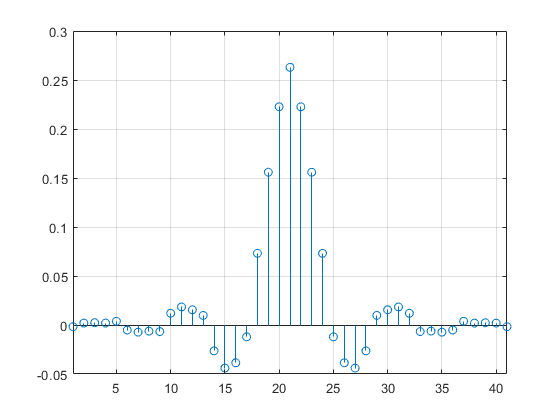Figure 8.  Interpolate-by-four filter coefficients.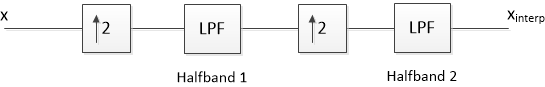Figure 9.  Interpolation by four using two Interpolate-by-two stages.

## References

1. Lyons, Richard G., Understanding Digital Signal Processing, 3rd Ed., Prentice-Hall, 2011, section 10.4.
2. The Mathworks website, https://www.mathworks.com/help/signal/ref/chebwin.html
3. Wikipedia, “Window Function”, https://en.wikipedia.org/wiki/Window_function
4. Robertson, Neil, “Simplest Calculation of Halfband Filter Coefficients”, https://www.dsprelated.com/showarticle/1113.php
5. Harris, Fredric J., Multirate Signal Processing for Communication Systems, Prentice Hall, 2004, Ch 7.

Neil Robertson               August, 2019

[ - ]
Comment by September 9, 2019Hi,

Thank you for this interesting post. I tried to implement it in python. If anyone was interested just look at this link: https://colab.research.google.com/github/Strato75/.... Please, let me know for bugs or any other issue.

Giovanni

[ - ]
Comment by September 9, 2019Giovanni,

Thanks for the Python code.

Neil

[ - ]
Comment by September 9, 2019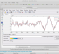Off the topic a little bit but this Python & math and dsp packages combined with Jupyter notebooks / Google co-lab type running environments is really a big power and facility..

Thanks for the code

[ - ]
Comment by September 12, 2019Hi Neil,

Thanks for the interesting article. I have succesfully run the Matlab code in Octave ver 5.1, by simply replacing the firpm function with the remez function.

remez is contained within the 'signal' add-on package for Octave.

[ - ]
Comment by September 12, 2019Thanks.  It certainly confused matters when Matlab changed the name of their Parks-McClellan function from remez to firpm.

[ - ]
Comment by September 14, 2019Hi,

I want to simulate a bidirectional full-duplex communication network and I don’t know how to go about it in MATLAB. Please do you have material which could be of help. Thank you

[ - ]
Comment by May 4, 2021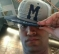Thank you very much for the article.

I was trying to implement a Farrow structure using Lagrange interpolation. The output signal's (the interpolated signal) spectrum was compressed as if the fundamental tone was close to zero. Also, there were some spurs that could be seen from fundamental frequency till fs/2.

Is it true that after interpolation, the spectrum will be compressed in the frequency domain?

[ - ]
Comment by May 4, 2021Hi Daniel,

No, the frequency at the output should be the same as that at the input.  Note, however, that for a sine of frequency f0, if you interpolate by two, f0/fs_out is 1/2 of f0/fs_in, where fs_in is input sample rate and fs_out is output sample rate.  For example, if f0 = 5 Hz, fs_in= 20 Hz, and fs_out= 40 Hz, then f0/fs_in = 1/4 and f0/fs_out= 1/8.

There is a discussion of Farrow interpolators in Michael Rice's book "Digital Communications, A Discrete-Time Approach".

regards,

Neil

[ - ]
Comment by May 6, 2021Thank you for the response and for the book.

The problem is that when representing the PSD, the fundamental frequency is very close to zero. and I suspect I am doing something wrong about that or it is the nature of the interpolation.

Also, I cannot detect the spurs of the signal in low frequencies(maybe I am trying to find out the SFDR). since my PSD representation is not perfect the spurs should really show up to be able to detect them using the findpeak function of Matlab.

with regards,

To post reply to a comment, click on the 'reply' button attached to each comment. To post a new comment (not a reply to a comment) check out the 'Write a Comment' tab at the top of the comments.